# 1. 算法的概念；

• 解决特定问题求解步骤的描述，在计算机中表现为指令的有限序列，并且每条指令表示一个或多个操作。
• 一个问题可以有多种算法，每种算法都有不同的效率。比如：1 + 2 + 3 + 4 + … + n 的值是多少，可以一个数字一个数字加，也可以用公式 (1 + n) * n / 2
• 一个算法具有五个特征：有穷性（能够计算完，不能无限计算下去，会变成死循环）、确切性（每一步都要有意义）、输入项（1 加到 100）、输出项（最终要给出一个结果）、可行性（算法要可以执行，每一步都是正确的）

# 2. 时间复杂度和空间复杂度；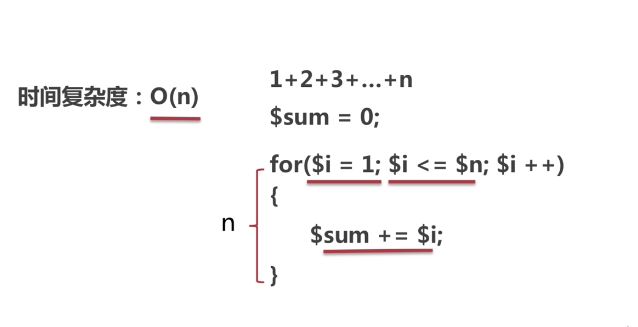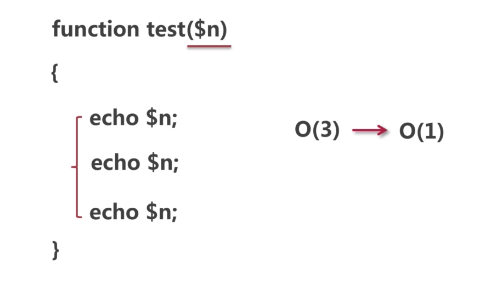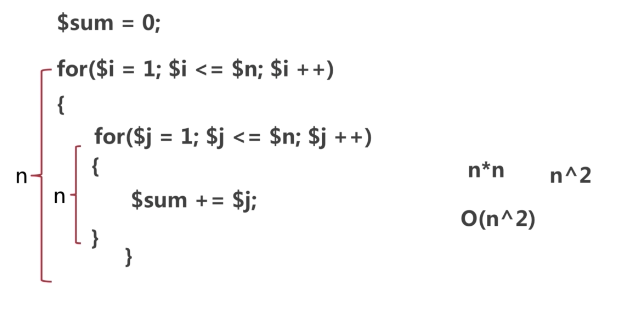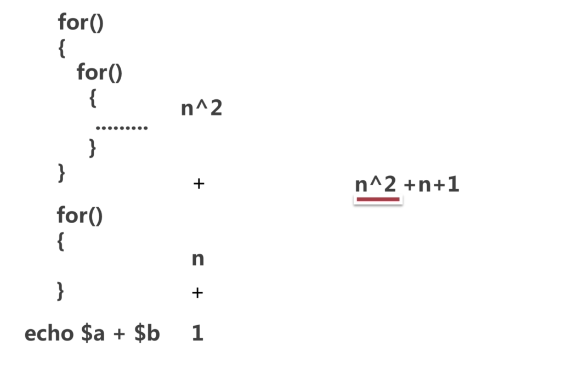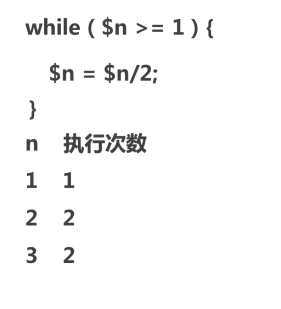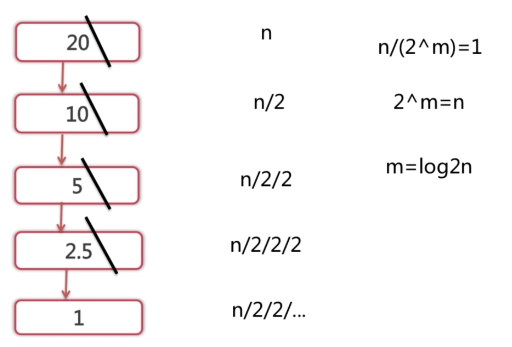# 3. 常见排序算法：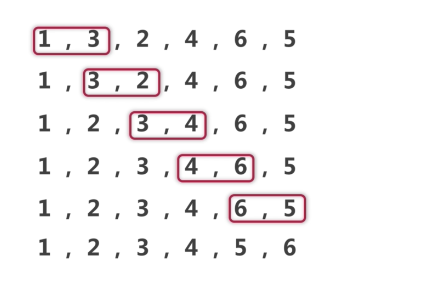// 实例：
<?php
$arr = [1, 3, 2, 4, 6, 5]; echo '<pre>'; for($i = 0, $c = count($arr); $i <$c; $i++){ for($j = $i+1;$j < $c;$j++){
if($arr[$i] > $arr[$j]){
$temp =$arr[$i];$arr[$i] =$arr[$j];$arr[$j] =$temp;
// 打印当前步骤的排序结果
print_r($arr); } } } // 最终排序结果 echo '<hr>'; print_r($arr);
exit;


// 实例：
<?php
$arr = [1, 3, 2, 4, 6, 5]; echo '<pre>'; // 数组中的第一个元素作为一个已经存在的有序表 for($i = 1; $i < count($arr); $i++){$temp = $arr[$i];
for($j =$i-1; $j >= 0 &&$arr[$j] >$temp; $j--){$arr[$j+1] =$arr[$j]; // 记录后移 }$arr[$j+1] =$temp;	// 插入到正确的位置
}
echo '<hr>';
print_r($arr); exit;  选择排序：每次从待排序的数据元素中选出最小（或最大）的一个元素，存放在序列的起始位置，直到全部待排序的数据元素排完 时间复杂度：最差 O(n 2)，平均 O(n 2) 空间复杂度：O(1) <?php$arr = [1, 3, 2, 4, 6, 5];

echo '<pre>';
for($i = 0,$c = count($arr);$i < $c;$i++){
// 记录第 $i 个元素后的所有元素最小值下标$min = $i; for($j = $i+1;$j < $c;$j++){
if($arr[$j] < $arr[$min]){
$min =$j;
}
}
$temp =$arr[$i];$arr[$i] =$arr[$min];$arr[$min] =$temp;
}
echo '<hr>';
print_R($arr); exit;  快速排序：通过一趟排序将套排序的数据分割成独立的两部分，其中一部分的所有数据都比另外一部分的所有数据要小，然后再按照此方法对这两部分数据分别进行快速排序，整个排序过程可以递归完成。 时间复杂度：最差 O(n 2)，平均 O(n*log2n) 空间复杂度：最差 O(n)，平均 O(log2n) <?php$arr = [1, 3, 2, 4, 6, 5];

$left = 0;$right = count($arr) - 1; function quick_sort(&$arr, $left,$right){
if($left >=$right)	return;

$temp =$arr[$left];$i = $left;$j = $right; while($i < $j){ while($i < $j &&$arr[$j] >=$temp){
$j--; } while($i < $j &&$arr[$i] <=$temp){
$i++; }$itemp = $arr[$i];
$arr[$i] = $arr[$j];
$arr[$j] = $itemp; } if ($left < $i){$arr[$left] =$arr[$i];$arr[$i] =$temp;
}
quick_sort($arr,$left, $i-1); quick_sort($arr, $i+1,$right);
}

quick_sort($arr,$left, $right); echo '<pre>'; print_r($arr);
exit;


• 总结：快速排序、归并排序的理想时间复杂度都是 O(n*log2n)，但是快速排序的时间复杂度并不稳定，最坏情况下复杂度为 O(n2) ，所以最理想的的算法还是归并排序

# 4. 常见查找算法。

• 总结：二分查找法的时间复杂度是最差 O(log2n)，顺序查找的时间复杂度最差为 O(n)，所以二分查找法更快，但是递归情况下，二分查找更消耗内容，时间复杂度为 O(log2n)

• 时间复杂度是描述算法时间消耗的计算量，空间复杂度就是内存的计算量
1. 对无序数组排序，最优的时间复杂度是什么，用 PHP 给出一个实际的例子（如了解，给出算法名称），该算法的空间复杂度是什么？
• 快速排序，也可以归并。
1. 一个有序数组中，查询待定的 item 是否存在的最优算法是什么？时间复杂度是什么？
• 二分查找法。

09-1104-063万+
10-183257
05-144万+
01-201万+
10-059716
06-072万+
10-14
08-071万+
06-1013万+
01-0712万+
07-192万+
05-2910万+
02-1750
09-21
09-281188
11-081万+
10-084717
08-302508